## Thursday, 29 September 2016

### For a Few Birthdays MoreOkay, maybe it CAN be worse than Paint Your Wagon.

It's that time again. Time to solve some math problem involving birthdays. I've previously solved the Classic Birthday Problem, as well as the Near-Match, First Match (and First Near-Match), Same Birthday as You, and Matched Couples (and larger groupings) variations.

I'm going to call this the Cover All Birthdays variation. We're looking for the probability that a group of people has every day of the year covered for birthdays.

Let's start by looking at a simpler problem. Suppose that we're tossing rings at some pegs. In this scenario we're assuming that every peg has an equal probability of being hit and that every ring must land on a peg. For any given numbers of n rings and k pegs, what's the probability that every peg is hit at least once? It is impossible to hit k pegs with fewer than k rings, so there is an implicit assumption in this analysis that n k.

With only one peg, the problem is trivial because all rings necessarily land on that one peg. There is only one possible outcome irrespective of n: the probability of hitting all pegs is 100%.

Since rings can land on any peg, including on the same peg multiple times, the total number of possible outcomes in this ring toss scenario is equal to k to the n-th power. To calculate the probability of hitting all pegs we need to count all of the ways that we could have at least one lone peg. So let's look at a two-peg situation now. There are 2 to the n-th power possible outcomes with the ring tosses. If we have one lone peg, that means there's just one peg left to hit. We already know that there's only one way to hit just one peg. But now, either peg could be the one that gets all the rings, so with 2 pegs there are 2*1 = 2 possible ways to have a lone peg. That means there are 2^n - 2 ways of hitting each peg at least once. The probability of covering all the pegs is therefore [2^n - 2] / [2^n] = 1 - 2^(1-n).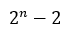Number of ways to throw n rings at 2 pegs, hitting all pegs at least once.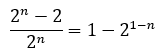Probability of hitting all pegs at least once when throwing n rings at 2 pegs.

What happens when we add in a third peg? Again, we could have all the rings land on one peg. This time there are 3 different pegs that could get all the rings, so there are 3 * 1 = 3 ways to hit only one peg. We already figured out that there were 2^n - 2 ways of covering 2 pegs, but now there are three pegs we could choose the two from, or C(3,2) = 3 ways to pick two out of three pegs. That gives us a total of 3 * (2^n - 2) ways of covering exactly two out of three pegs. We're left with 3^n - 3*(2^n-2) - 3 ways of covering all the pegs, out of a total 3^n permutations. Therefore, the probability of covering all three pegs is: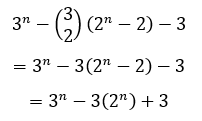Number of ways to throw n rings at 3 pegs, hitting all pegs at least once.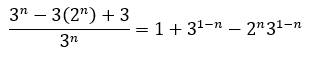Probability of hitting all pegs at least once when throwing n rings at 3 pegs.

Let's now define a function F[n,k] as the number of ways of hitting each of the k pegs at least once from n tosses. That recursive relationship looks like this:Recursive relationship defining the number of ways you can throw n rings hitting all k pegs at least once.Probability of throwing n rings and hitting all k pegs at least once.

As you keep adding pegs you'll see that the equations quickly start to get lengthy. Using the recursive relationship we could take a brute force calculation approach to evaluate F[n,k], but that quickly gets to be very inefficient as k increases. We'd like a different form of the equation. If we expand and simplify for k = 4 and k = 5, we get the following: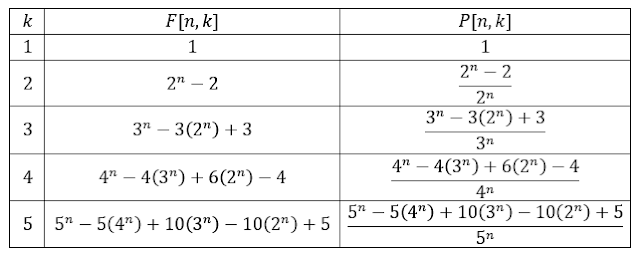Expanded and simplified expressions for F[n,k] and P[n,k] for k = 1 to 5.

Just for fun, let's plot those out as well so you can see how many tosses you need to have a reasonable chance of hitting all of the pegs.Tabulated values of the probability of throwing n rings and hitting all k pegs at least onceGraph showing the probability of hitting all pegs at least once with n tosses.

In order to have at least 50% chance of hitting all of the pegs, you need only 2 tosses when there are 2 pegs, 5 tosses for 3 pegs, 7 tosses for 4 pegs, and 10 tosses for 5 pegs.

Now, going back to the expanded equations we wrote out, you might've spotted a pattern. We can define F[n,k] in closed-form using binomial coefficients and summation notation as follows:Solution to the recursive relation for F[n,k].

Okay, so how does this relate to the Cover All Birthdays problem? Well if instead of n rings we have n randomly selected people and instead of k pegs we have 365 possible days a person could be born on, the problem is modeled exactly the same way. There are 365^n permutations of birthdays in a group of n people and there are F[n,365] ways of covering each possibility at least once:Number of birthday combinations in a group of n people where every day of the year is covered at least once.

Dividing by 365^n to get the probability of covering all birthdays, we can make the following graph:Probability of covering all 365 birthdays in a group of randomly selected people.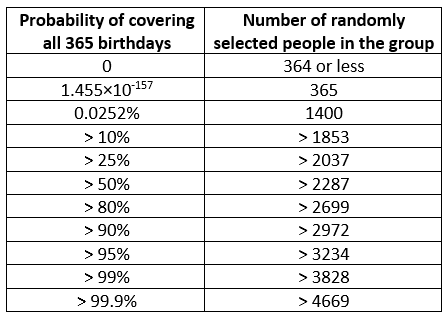Size of group required to achieve the given threshold probabilities of covering all 365 birthdays.

For n < 365 we know the probability of covering all the birthdays is exactly zero. For n = 365, there's less than 1 in 6.873×10¹⁵⁶ chance of covering every day of the year. If we double that to n = 730, we increase the probability by a factor of nearly 3.45×10¹²⁸, but that's still a negligibly small chance of about 1 in 1.993×10²⁸. Chances of covering all birthdays are vanishingly small until approximately n = 1400. We need at least n = 2287 to exceed a 50% chance of covering all birthdays and at least n = 3828 to give us better than a 99% chance.

1.2.